# Area of a Triangle

Go back to  'Coordinate-Geometry'

Consider the following three points:

$\begin{array}{l}A = \left( { - 2,\;1} \right)\\B = \left( {3,\;2} \right)\\C = \left( {1,\;5} \right)\end{array}$

If you plot these three points in the plane, you will find that they are non-collinear, which means that they can be the vertices of a triangle, as shown below: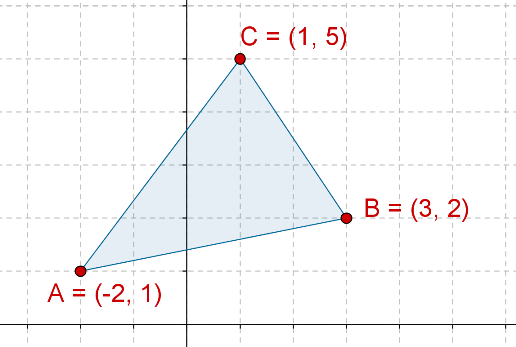The question which we now want to consider is: how can we calculate the area of this triangle using the coordinates of the vertices? Note that the area of any triangle is:

${\rm{Area}} = \frac{1}{2}\;bh$

So, one thing which we can do is to take one of the sides of the triangles as the base, and calculate the corresponding height, that is, the length of the perpendicular drawn from the opposite vertex to this base. You are urged to try and do that.

However, we want to do something better: rather than calculating the height, we want to use only the coordinates of the three vertices to calculate the area. To do that, consider the following figure carefully. In this figure, we have dropped perpendiculars AD, CF and BE from the vertices of our triangle to the horizontal axis. Notice that three trapeziums are formed: ACFD, BCFE and ABED.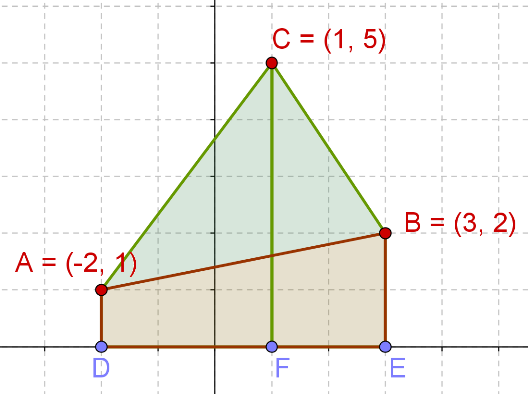Can we express the area of our triangle in terms of the areas of these three trapeziums? Yes, we can:

${\rm{Area}}\left( {{\rm{\Delta ABC}}} \right){\rm{ = }}\left\{ \begin{array}{l}{\rm{Area}}\;\left( {{\rm{Trap}}{\rm{.}}\;{\rm{ACFD}}} \right)\\ \,\,\,\,\,\,\,\,\,\,\,\, \,\,\,\,\,\,\,\,\,\,\,\,\,\,\,\,\,\,\,\,\,\,\,\,\,\,\,\,\,\,\,\,\, + \\{\rm{Area}}\;\left( {{\rm{Trap}}{\rm{.}}\;{\rm{BCFE}}} \right)\\ \,\,\,\,\,\,\,\,\,\,\,\,\,\,\,\,\,\,\,\,\,\,\,\,\,\,\,\,\,\,\,\,\,\,\,\,\,\,\,\,\,\,\,\,\,\, - \\{\rm{Area}}\;\left( {{\rm{Trap}}{\rm{.}}\;{\rm{ABED}}} \right)\end{array} \right.$

Now our task is simplified, because we know how to calculate the area of a trapezium in terms of the lengths of the parallel sides (the bases of the trapezium) and the distance between the parallel sides (the height of the trapezium):

${\rm{Trap}}{\rm{.}}\;{\rm{Area}} = \frac{1}{2} \times \;{\rm{Sum}}\;{\rm{of}}\;{\rm{bases}}\;{\rm{ \times }}\;{\rm{Height}}$

Consider any one trapezium, say ACFD. Its bases are AD and CF, and its height is DF. AD and CF can easily be seen to be the y coordinates of A and C, while DF is the difference between the x coordinates of C and A. Similarly, the bases and heights of the other two trapeziums can be easily calculated. Thus, we have:

\begin{align}&{\rm{Area}}\;\left( {{\rm{Trap}}{\rm{.}}\;{\rm{ACFD}}} \right) = \frac{1}{2} \times \left( {AD + CF} \right) \times DF\\&\qquad\qquad\qquad\qquad\quad= \frac{1}{2} \times \left( {1 + 5} \right) \times 3 = 9\\&{\rm{Area}}\;\left( {{\rm{Trap}}{\rm{.}}\;{\rm{BCFE}}} \right) = \frac{1}{2} \times \left( {CF + BE} \right) \times FE\\&\qquad\qquad\qquad\qquad\quad= \frac{1}{2} \times \left( {5 + 2} \right) \times 2 = 7\\&{\rm{Area}}\;\left( {{\rm{Trap}}{\rm{.}}\;{\rm{ABED}}} \right) = \frac{1}{2} \times \left( {AD + BE} \right) \times DE\\&\qquad\qquad\qquad\qquad\quad= \frac{1}{2} \times \left( {1 + 2} \right) \times 5 = \frac{{15}}{2}\end{align}

Finally, the area of our triangle can be calculated:

\begin{align}{\rm{Area}}\left( {\Delta {\rm{ABC}}} \right) &= 9 + 7 - \frac{{15}}{2}\\&=\frac{{17}}{2}\,{\rm{sq}}{\rm{.}}\,{\rm{units}}\end{align}

Let us now generalize this approach.

Consider three non-collinear points in the plane:

$\begin{array}{l}A = \left( {{x_1},\;{y_1}} \right)\\B = \left( {{x_2},\;{y_2}} \right)\\C = \left( {{x_3},\;{y_3}} \right)\end{array}$

If you plot these points, they will be the vertices of a triangle: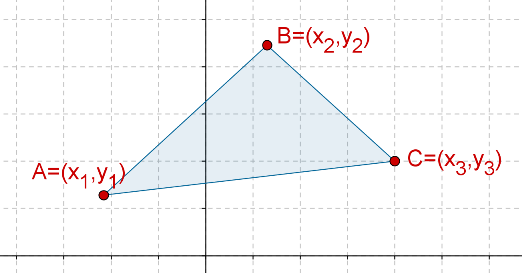Once again, we drop perpendiculars from A, B and C to the horizontal axis, as shown below: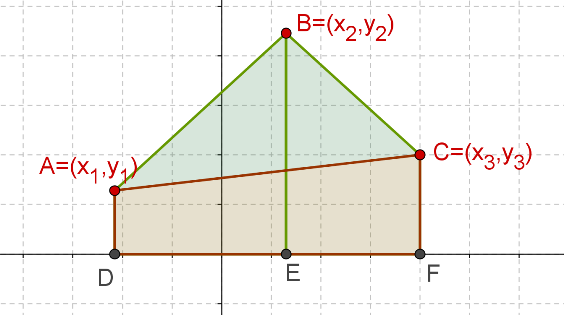Thus, we have:

${\rm{Area}}\;\left( {{\rm{\Delta ABC}}} \right){\rm{ = }}\left\{ \begin{array}{l}{\rm{Area}}\;\left( {{\rm{Trap}}{\rm{.}}\;{\rm{ABED}}} \right)\\\,\,\,\,\,\,\,\,\,\,\,\, \,\,\,\,\,\,\,\,\,\,\,\,\,\,\,\,\,\,\,\,\,\,\,\,\,\,\,\,\,\,\,\,\, + \\{\rm{Area}}\;\left( {{\rm{Trap}}{\rm{.}}\;{\rm{CBEF}}} \right)\\\,\,\,\,\,\,\,\,\,\,\,\, \,\,\,\,\,\,\,\,\,\,\,\,\,\,\,\,\,\,\,\,\,\,\,\,\,\,\,\,\,\,\,\,\,\,\, - \\{\rm{Area}}\;\left( {{\rm{Trap}}{\rm{.}}\;{\rm{ACFD}}} \right)\end{array} \right.$

Now, we calculate the area of each trapezium separately:

$\begin{array}{l}({\rm{i}}) & {\rm{Area}}\;\left( {{\rm{Trap}}{\rm{.}}\;{\rm{ABED}}} \right)\\ & = \frac{1}{2} \times \left( {AD + BE} \right) \times DE\\ & = \frac{1}{2} \times \left( {{y_1} + {y_2}} \right) \times \left( {{x_2} - {x_1}} \right) & ...({\rm{I}})\{\rm{ii}}) & {\rm{Area}}\;\left( {{\rm{Trap}}{\rm{.}}\;{\rm{CBEF}}} \right)\\ & = \frac{1}{2} \times \left( {BE + CF} \right) \times EF\\ & = \frac{1}{2} \times \left( {{y_2} + {y_3}} \right) \times \left( {{x_3} - {x_2}} \right) & ...({\rm{II}})\\({\rm{iii}})&{\rm{Area}}\left( {{\rm{Trap}}{\rm{.}}\;{\rm{ACFD}}} \right)\\ & = \frac{1}{2} \times \left( {AD + CF} \right) \times DF\\ & = \frac{1}{2} \times \left( {{y_1} + {y_3}} \right) \times \left( {{x_3} - {x_1}} \right) & ...({\rm{III}})\end{array}$ Next, we express the area of our triangle in terms of these areas: \begin{align}&{\rm{Area}}\;\left( {\Delta ABC} \right)\\&= \left( {\rm{I}} \right) + \left( {{\rm{II}}} \right) - \left( {{\rm{III}}} \right)\\& = \frac{1}{2}\left( {{y_1} + {y_2}} \right)\left( {{x_2} - {x_1}} \right)\\& + \frac{1}{2}\left( {{y_2} + {y_3}} \right)\left( {{x_3} - {x_2}} \right)\\&- \frac{1}{2}\left( {{y_1} + {y_3}} \right)\left( {{x_3} - {x_1}} \right) & ...({\rm{IV}})\end{align} This is the expression for the area of the triangle in terms of the coordinates of its vertices. However, we should try to simplify it a bit so that it is easy to remember. For that, we write out the product of the two brackets in each of the three terms above: $\begin{array}{l}\left( {{y_1} + {y_2}} \right)\left( {{x_2} - {x_1}} \right)\\ \,\,\,\,\,\;\;\;\;\; \;\;\;\;\; = {x_2}{y_1} - {x_1}{y_1} + {x_2}{y_2} - {x_1}{y_2}\\\left( {{y_2} + {y_3}} \right)\left( {{x_3} - {x_2}} \right)\\ \,\,\,\,\,\;\;\;\;\; \;\;\;\;\; = {x_3}{y_2} - {x_2}{y_2} + {x_3}{y_3} - {x_2}{y_3}\\\left( {{y_1} + {y_3}} \right)\left( {{x_3} - {x_1}} \right)\\\,\,\,\,\,\;\;\;\;\; \;\;\;\;\; = {x_3}{y_1} - {x_1}{y_1} + {x_3}{y_3} - {x_1}{y_3}\end{array}$ Thus, $\begin{array}{l}{\rm{Area}}\;\left( {\Delta ABC} \right)\\ = \frac{1}{2}\left( \begin{array}{l} - {x_1}{y_2} + {x_1}{y_3} + {x_2}{y_1}\\ - {x_2}{y_3} + {x_3}{y_2} - {x_3}{y_1}\end{array} \right)\\ = \frac{1}{2}\left\{ \begin{array}{l}{x_1}\left( {{y_3} - {y_2}} \right) + \\{x_2}\left( {{y_1} - {y_3}} \right) + {x_3}\left( {{y_2} - {y_1}} \right)\end{array} \right\}\\ = - \frac{1}{2}\left\{ \begin{array}{l}{x_1}\left( {{y_2} - {y_3}} \right) + \\{x_2}\left( {{y_3} - {y_1}} \right) + {x_3}\left( {{y_1} - {y_2}} \right)\end{array} \right\}\end{array}$ This is a very symmetric expression, and there is a very easy technique to remember it, which we will now discuss. Write the coordinates as follows, in a grid fashion (the third row as constant entries): $\begin{array}{l}{x_1} & & {x_2} & & {x_3}\\{y_1} & & {y_2} & & {y_3}\\1 & & 1 & & 1\end{array}$ Let us call this grid the coefficients grid, or CG for short. Now, the first term in the expression for the area is \({x_1}\left( {{y_2} - {y_3}} \right). To write this, we ignore the terms in the first row and column of the CG, and proceed according to the following visual representation (the cross arrows represent multiplication):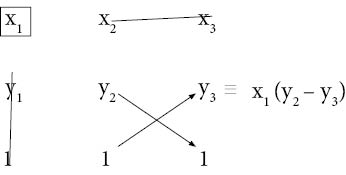The second term in the expression for the area is $${x_2}\left( {{y_3} - {y_1}} \right)$$ . To write this, we ignore the terms in the first row and second column of the CG, but this time we reverse the order, that is, we have $${y_3} - {y_1}$$ instead of $${y_1} - {y_3}$$: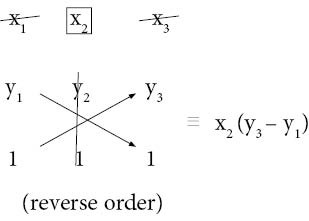Next, the third term in the expression for the area is $${x_3}\left( {{y_1} - {y_2}} \right)$$ . To write this, we ignore the terms in the first row and third column of the CG: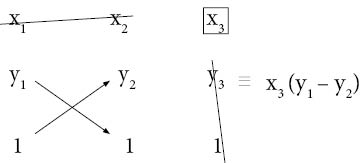Finally, we add these three terms to get the area (and divided by a factor of 2, because we had this factor in the original expression we determined):

$A = \frac{1}{2}\left| \begin{array}{l}{x_1}\left( {{y_2} - {y_3}} \right) + {x_2}\left( {{y_3} - {y_1}} \right)\\ \;\;\;\;\;\;\;\;\;\;\;\;\;+ {x_3}\left( {{y_1} - {y_2}} \right)\end{array} \right|$

Note that we have put a modulus sign (vertical bars) around our algebraic expression, and removed the negative sign we obtained in the original expression. We did this only because we eventually want a positive value for the area. So even if we get a negative value through the algebraic expression, the modulus sign will ensure that it gets converted to a positive value. We can write the above expression for area compactly in terms of the CG as follows:

$A = \frac{1}{2}\;\left| {\begin{array}{*{20}{c}}{{x_1}}&{{x_2}}&{{x_3}}\\{{y_1}}&{{y_2}}&{{y_3}}\\1&1&1\end{array}} \right|$

Example 1: Consider a triangle with the following vertices:

$\begin{array}{l}A = \left( { - 1,\;2} \right)\\B = \left( {2,\;3} \right)\\C = \left( {4,\; - 3} \right)\end{array}$

What is the area of this triangle?

Solution: To illustrate the use of the coefficients grid or CG, we will calculate each of the three terms in the formula for the area separately, and then put them together to obtain the final value.

The CG is:

$\left| {\begin{array}{*{20}{c}}{ - 1}&2&4\\2&3&{ - 3}\\1&1&1\end{array}} \right|$

### First Term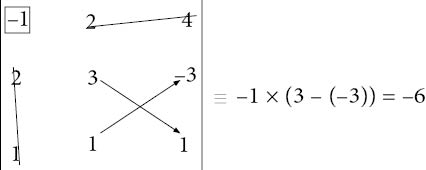### Second Term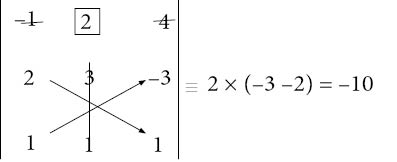### Third Term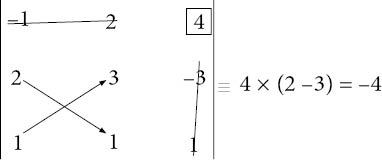Finally, we put these three values together, taking care to not ignore the factor of 2, and also to use the modulus sign to get a positive value:

\begin{align}&{\rm{Area}}\;\left( {\Delta ABC} \right)\\ &= \frac{1}{2}\left| {\left( { - 6} \right) + \left( { - 10} \right) + \left( { - 4} \right)} \right|\\ &= \frac{1}{2} \times 20\\ &= 10\;{\rm{sq}}{\rm{.}}\;{\rm{units}}\end{align}

You may be wondering about one thing: why did we not ask you to remember the area formula directly (as done normally in textbooks), and why did we make use of this coefficients grid? The answer is simple: using the CG, the chances of making a mistake are lesser, and more importantly, these kinds of number grids are actually very important mathematical entities called determinants, which you will study detail in higher classes. However, it’s a good thing to get familiar with the basic idea right now.

Example 2: Find the area of a triangle with the following vertices:

$\begin{array}{l}A\left( {3,\;4} \right)\\B\left( {4,\;7} \right)\\C\left( {6,\; - 3} \right)\end{array}$

Solution: We have:

\begin{align}&{\rm{Area}} = \frac{1}{2}\left| {\,\begin{gathered}{}3&4&6\\4&7&{ - 3}\\1&1&1\end{gathered}\,} \right|\;\begin{gathered}{} \leftarrow &{x\;{\rm{row}}}&{}\\ \leftarrow &{y\;{\rm{row}}}&{}\\ \leftarrow &{{\rm{constant}}}&{}\end{gathered}\\&\qquad= \frac{1}{2}\;\left| \begin{array}{l}3 \times \left( {7 - \left( { - 3} \right)} \right) + 4 \times \left( { - 3 - 4} \right)\\ + 6\left( {4 - 7} \right)\end{array} \right|\\&\qquad = \frac{1}{2}\;\left| {30 - 28 - 18} \right|\, = \frac{1}{2} \times 16 = 8\;{\rm{sq}}{\rm{.}}\;{\rm{units}}\end{align}

Coordinate Geometry
Grade 9 | Questions Set 1
Coordinate Geometry
Coordinate Geometry
Grade 10 | Questions Set 1
Coordinate Geometry